Test: Geometry- 3

# Test: Geometry- 3

Test Description

## 15 Questions MCQ Test | Test: Geometry- 3

Test: Geometry- 3 for Quant 2023 is part of Quant preparation. The Test: Geometry- 3 questions and answers have been prepared according to the Quant exam syllabus.The Test: Geometry- 3 MCQs are made for Quant 2023 Exam. Find important definitions, questions, notes, meanings, examples, exercises, MCQs and online tests for Test: Geometry- 3 below.
Solutions of Test: Geometry- 3 questions in English are available as part of our course for Quant & Test: Geometry- 3 solutions in Hindi for Quant course. Download more important topics, notes, lectures and mock test series for Quant Exam by signing up for free. Attempt Test: Geometry- 3 | 15 questions in 15 minutes | Mock test for Quant preparation | Free important questions MCQ to study for Quant Exam | Download free PDF with solutions
 1 Crore+ students have signed up on EduRev. Have you?
Test: Geometry- 3 - Question 1

### The line AB is 6 m, in length and is tangent to the inner of the two concentric circles at point C. It is known that the radii of the two circles are integers. The radiusof the outer circle is-------, where A and B are points on the outer circle.

Detailed Solution for Test: Geometry- 3 - Question 1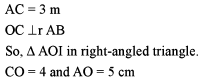Test: Geometry- 3 - Question 2

### Two chords of lengths a and b of a circle subtend 60° and 90° angles at the centre respectively. Which of the following is correct?

Detailed Solution for Test: Geometry- 3 - Question 2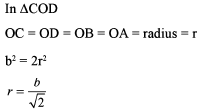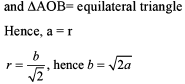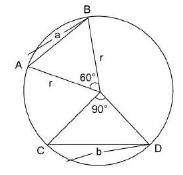Test: Geometry- 3 - Question 3

### A chord of length 32 cm is placed inside a circle of radius 20 cm and a point whose distance from the centre of the circle is 13 cm, is marked on the chord. Calculate the lengths of the segment of the chord.

Detailed Solution for Test: Geometry- 3 - Question 3

AQ = (16 + 5 = 21) cm and QB = 32 - 21 = 11 cm

Test: Geometry- 3 - Question 4

The biggest possible regular hexagon H is cut out of an equilateral triangle X. The biggest possible equilateral triangle Y is cut out from the hexagon H. What is the ratio of the areas of the equilateral triangles X and Y?

Detailed Solution for Test: Geometry- 3 - Question 4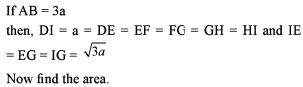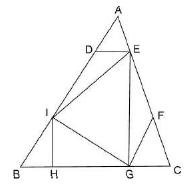Test: Geometry- 3 - Question 5

In any quadrilateral ABCD, the diagonal AC and BD intersect at a point X. If E, F, G and H are the midpoints of AX, BX, CX and DX respectively, then what is the ratio of (EF + FG + GH + GE) to (AD + DC + CB + BA)?

Detailed Solution for Test: Geometry- 3 - Question 5

A midpoint is a point on a line segment equally distant from the two endpoints. The Midpoint Theorem is used to make a bold statement regarding triangle sides and their lengths. Given a triangle, if we connect two sides with a line segment, and this line segment joins each of the two sides at the centers, or midpoints of each side, we can know two very important aspects about the triangle and the relationships between the sides.

Test: Geometry- 3 - Question 6

One of the angles of a parallelogram is of 150°. Altitudes are drawn from the vertex of this angle. If these altitudes measure 6 cm and 8 cm, then find the perimeter of the parallelogram.

Detailed Solution for Test: Geometry- 3 - Question 6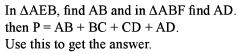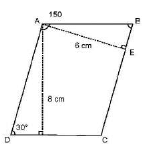Test: Geometry- 3 - Question 7

Let C1 and C2 be the inscribed and circumscribed circles of a triangle with sides 3 cm, 4 cm and 5 cm.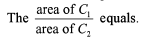Detailed Solution for Test: Geometry- 3 - Question 7

r1 = 2.5 cm

r = 1 cm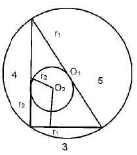Test: Geometry- 3 - Question 8

PQRS is a trapezium with PQ and RS parallel. PQ = 6 cm, QR = 5 cm, RS = 3 cm, PS = 4 cm. The area of PQRS is

Detailed Solution for Test: Geometry- 3 - Question 8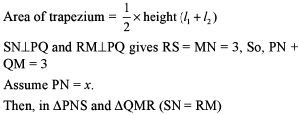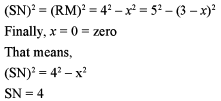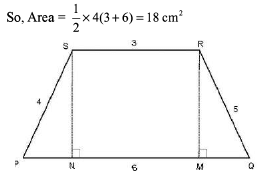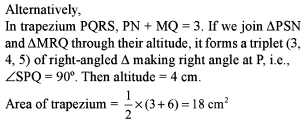Test: Geometry- 3 - Question 9

Based on the figure below, what is the value of x, if y=10.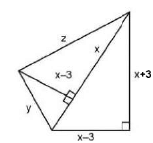Detailed Solution for Test: Geometry- 3 - Question 9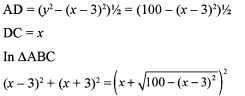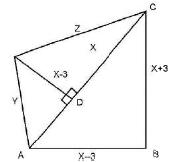Now, putting the values of the given options, we can calculate the answer. It is none of these.
Alternatively, this question can be done very easily using the options.

Test: Geometry- 3 - Question 10

A ball of diameter 15 cm is floating so that the top of the ball is 5 cm above the smooth surface (water) of the pond. What is the circumference in centimeters of the circle formed by the contact of the water surface with the ball?

Detailed Solution for Test: Geometry- 3 - Question 10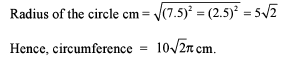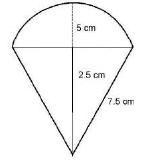Test: Geometry- 3 - Question 11

Two circles APQC and PBDQ intersect each other at the points P and Q and APB and CQD are two parallel straight lines. Then only one of the following statements is always true. Which one is it?

Detailed Solution for Test: Geometry- 3 - Question 11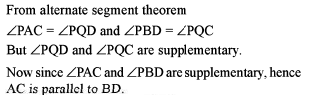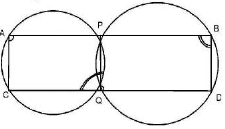Test: Geometry- 3 - Question 12

Three horses are grazing within a semi-circular field. In the diagram given below, AB is the diameter of the semi-circular field with centre at O. Horses are tied up at P, R and S such that PO and RO are the radii of semi-circles with centres at P and R respectively and S is the centre of the circle touching the two semi-circles with diameters AO and OB. The horses tied at P and R can graze within the respective semi-circle and the horse tied at S can graze within the circle centered at S. The percentage of the area of the semi-circles with diameter AB that cannot be grazed by the horses is nearest to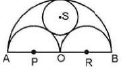Detailed Solution for Test: Geometry- 3 - Question 12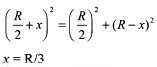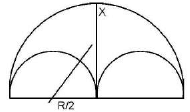Test: Geometry- 3 - Question 13

Consider the following figure: AB = 10 cm, AC = 17 cm, BC = 21 cm and EHFD is a square. Find the length of the side of square (in cm).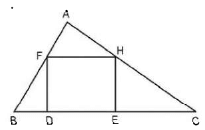Detailed Solution for Test: Geometry- 3 - Question 13

Side of the square should always be less than the attitude of the triangle .

Test: Geometry- 3 - Question 14

For a regular octagon inscribed in a circle of radius 1 cm, the product of the distance from a fixed vertex to the other seven vertices is

Detailed Solution for Test: Geometry- 3 - Question 14

We have to calculate AH x AG x AF x AE x AD x AC x AB

AE = 2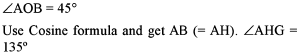A gain by C osine form ula get A G (= A C) and A F (= AD)

Test: Geometry- 3 - Question 15

A ring of diameter 10 cm is suspended from a point 12 cm vertically above the centre by six equal strings. The strings are attached to the circumference of the ring at equal intervals, thus keeping the ring in a horizontal plane. The value of cosine of the angle between the two adjacent strings lies in between

Detailed Solution for Test: Geometry- 3 - Question 15

Consider the problem as a pyramid to the base of a regular hexagon.
Now in A POR

OP = 12 cm

OR = 5 cm

PR = 13 cm

Now cosine formula will give the answer.

Information about Test: Geometry- 3 Page
In this test you can find the Exam questions for Test: Geometry- 3 solved & explained in the simplest way possible. Besides giving Questions and answers for Test: Geometry- 3, EduRev gives you an ample number of Online tests for practice(Scan QR code)